# Hausdorffness is hereditary

Jump to: navigation, search
This article gives the statement, and possibly proof, of a topological space property (i.e., Hausdorff space) satisfying a topological space metaproperty (i.e., subspace-hereditary property of topological spaces)
View all topological space metaproperty satisfactions | View all topological space metaproperty dissatisfactions
Get more facts about Hausdorff space |Get facts that use property satisfaction of Hausdorff space | Get facts that use property satisfaction of Hausdorff space|Get more facts about subspace-hereditary property of topological spaces

## Statement

Any subspace of a Hausdorff space is Hausdorff in the subspace topology.

## Definitions used

### Hausdorff space

Further information: Hausdorff space

A topological space$X$ is Hausdorff if given distinct points$a,b \in X$ there exist disjoint open subsets$U,V$ containing$a,b$ respectively.

### Subspace topology

Further information: subspace topology

If$A$ is a subset of$X$, we declare a subset$V$ of$A$ to be open in$A$ if$V = U \cap A$ for an open subset$U$ of$X$.

## Proof

Given: A topological space$X$, a subset$A$ of$X$. Two distinct points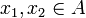$x_1,x_2 \in A$.

To prove: There exist disjoint open subsets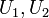$U_1,U_2$ of$A$ such that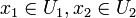$x_1 \in U_1,x_2 \in U_2$.

Proof:

Step no. Assertion/construction Facts used Given data used Previous steps used Explanation
1$x_1, x_2$ are distinct points of$X$$x_1,x_2$ are distinct points of$A$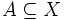$A \subseteq X$
2 There exist disjoint open subsets$V_1, V_2$ of$X$ such that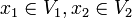$x_1 \in V_1, x_2 \in V_2$.$X$ is Hausdorff Step (1) Step-given direct
3 Define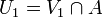$U_1 = V_1 \cap A$ and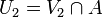$U_2 = V_2 \cap A$.
4$U_1, U_2$ are open subsets of$A$. definition of subspace topology Steps (2), (3) By Step (2),$V_1,V_2$ are open, so by the definition of subspace topology,$U_1, U_2$ are open as per their definitions in Step (3).
5$U_1, U_2$ are disjoint. Steps (2), (3) follows directly from$V_1,V_2$ being disjoint
6$x_1 \in U_1, x_2 \in U_2$$x_1,x_2 \in A$ Steps (2), (3) By Step (3),$U_1 = V_1 \cap A$. By Step (2),$x_1 \in V_1$, and we are also given that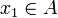$x_1 \in A$, so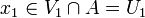$x_1 \in V_1 \cap A = U_1$. Similarly,$x_2 \in U_2$.
7$U_1,U_2$ are the desired open subsets of$A$. Steps (4)-(6) Step-combination direct, it's what we want to prove.
This proof uses a tabular format for presentation. Learn more about tabular proof formats|View all pages on facts with proofs in tabular format